# Spectral geometry

To any Riemannian manifoldone can associate a number of natural elliptic differential operators which arise from the geometric structure of. Usually, these operators act in the spaceof smooth sections of some vector bundleoverequipped with a positive-definite Riemannian inner product. Ifis a complete Riemannian manifold (cf. Complete Riemannian space), then many of these operators give rise to self-adjoint operators (cf. also Self-adjoint operator) in the Hilbert space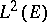of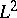-sections of. Examples of such operators are the Hodge–de Rham Laplacians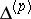(cf. also Laplace operator) acting on the space of differential-forms on,(forthis is just the Laplace–Beltrami operatoracting on the space of smooth functions on; cf. also Laplace–Beltrami equation) and, more generally, second-order self-adjoint operators of Laplace type on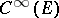, that is, with leading symbol given by the metric tensor.

Spectral geometry deals with the study of the influence of the spectra of such operators on the geometry and topology of a Riemannian manifold (possibly with boundary; cf. also Spectrum of an operator). Everything started with the classical Weyl asymptotic formula, and was later translated into the colloquial question "Can one hear the shape of a manifold?" by several authors, most notably M. Kac [a12], because of the analogy with the wave equation.

The case which has been studied most is that ofon a compact Riemannian manifold, perhaps with boundary. When, one imposes Dirichlet or Neumann boundary conditions to get a self-adjoint extension. Then the corresponding self-adjoint extension has countably many eigenvalues and these form a sequence(eachbeing repeated with its multiplicity), which accumulate only at infinity, see [a6]. The entire collection of's with their finite multiplicities is called the spectrum ofand is denoted by. Two compact Riemannian manifolds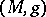and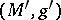are said to be isospectral if. Two isometric compact Riemannian manifolds are necessarily isospectral. The inverse problem, namely to what extent does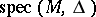determine, up to isometry (see also below), has been studied quite extensively. In particular, the answer to the question whether isospectral Riemannian manifolds are necessarily isometric is now (1998) well known to be negative. The first counterexample was a pair of isospectral-dimensional flat tori given by J. Milnor in 1964. Until 1980, however, the only other examples discovered were a few additional pairs of flat tori or twisted products with tori. Starting in 1980, many examples as well as fairly general techniques for constructing examples of isospectral Riemannian manifolds that are not isometric have appeared, see [a2], [a4], [a9], [a10], [a11], [a13], [a23], and [a19]. Among these examples are pairs of manifolds with non-isomorphic fundamental groups, locally symmetric spaces both of rank one and higher rank, Riemann surfaces of every genus, continuous families of isospectral Riemannian manifolds, lens spaces, non-locally isometric Riemannian manifolds and other examples. T. Sunada introduced a systematic method for constructing pairs of non-isometric isospectral Riemannian manifolds (see [a22]), and H. Pesce succeeded in giving a major strengthening of this method (see [a20], [a21]).

Since it is difficult to studydirectly, instead one introduces certain functions of the eigenvalues which can be used to extract geometric information from the spectrum. Some useful such functions having interesting applications to spectral geometry are discussed below.

### Heat coefficients.

The connecting link between the heat equation approach to index theory and spectral geometry is the asymptotic expansion of the heat kernel. For simplicity, assume that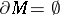. S. Minakshisundaram and A. Pleijel have proved that for every closed Riemannian manifoldthere exists an asymptotic expansionas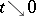. The numerical invariants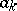are the heat coefficients and they determine and are uniquely determined by. The numbersare locally computable from the metric. In fact, they are universal polynomials in the curvature of the Levi-Civita connection associated toand their covariant derivatives. In particular, it follows from this expansion thatdetermines,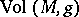and the total scalar curvature of(and hence, by the Gauss–Bonnet theorem, the Euler characteristic ofifis a surface). There are more results along these lines; for instance, the fact that standard spheres and real projective spaces in dimensions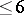are characterized by their spectra can be deduced from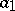,,. The's get more and more complicated asincreases, but at least the leading terms (i.e. the terms with a maximal number of derivatives) in thefor allcan be described. General references for this area are [a1], [a3], [a7], [a8], and [a14].

### The regularized determinant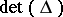of.

This regularized determinant is defined bywhere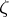is the meromorphic extension toof the zeta-function associated to the non-zero eigenvalues. It is a global spectral invariant, that is, it cannot be computed locally from the metric. There are very interesting results concerning the extremal points ofas a function of the metricon a given closed surface. References are [a17], [a16] and [a18].

### The regularized characteristic determinantof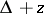.

This regularized characteristic determinant is defined by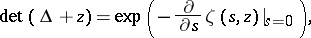whereis the analytic continuation to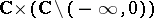of the function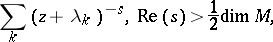where the sum ranges over the non-zero eigenvalues. This function generalizes the concept of a characteristic polynomial in finite dimension. For a closed surfaceof constant negative curvature,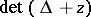is closely related to the Selberg zeta-function. A reference is [a5].

### Topological aspects.

If one takes into account the spectra of other natural geometric operators, then global topological aspects come into play. For instance, a linear combination of the values at zero of the analytic continuations toof the zeta-functions associated respectively to the non-zero eigenvalues of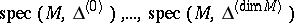gives the Euler characteristic ofand another linear combination of the derivatives at zero of these functions gives the Reidemeister torsion. A general reference for this area as well as for spectral problems on non-compact Riemannian manifolds is [a15].